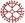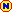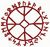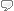# drawdown

## Norwegian translation: tap

GLOSSARY ENTRY (DERIVED FROM QUESTION BELOW)
 English term or phrase: drawdown Norwegian translation: tap Entered by:

 04:09 Feb 21, 2005
English to Norwegian translations [PRO]
Bus/Financial - Finance (general)
 English term or phrase: drawdown Maximum drawdown Uninterrupted drawdown From info on Hedge Fund
 Helene Olsen RichardsUnited StatesLocal time: 05:17
 tap Explanation:http://www.andreassteiner.net/performanceanalysis/?Risk_Meas... The maximum drawdown can be loosely defined as the largest drop from a peak to a bottom in a certain time period. Maximum drawdown captures a path-dependant feature of a time series which is not represented in the histogram of the return time series. Given a time series with N log returns r(t) over t = 1 to T, we can define... Maximum Loss = min [r(1), r(1)+r(2), r(1)+r(2)+r(3), ..., r(1)+...+r(N)] = min [ sum [r(i)] ] t=0..N i=1..t In words: The minimum cummulated return from the beginning in a certain time period. Drawdown = max [ sum[r(t)] ] - r(t) t = 0..T Maximum Drawdown = min [r(1), r(1)+r(2), r(1)+r(2)+r(3), ..., r(1)+...+r(N), r(2), r(2)+r(3), r(2)+r(3)+r(4), ..., r(2)+...+r(N), ..., r(N)] = min [ sum [r(j)] ] i=1..t, t=1..N j=i..t In words: The minimum cummulated return from any beginning points over a certain time period. = max [ Drawdown(t) ] t=0..T ...the last formula yields the end-point of the maximum drawdown period. The starting point is found at the last time point Drawdown(t) was equal to zero. Maximum drawdown is always smaller than or equal to the difference between maximum loss and maximum gain. Maximum loss & gain are the global extreme values, maximum drawdown is a concept base on the local minimum of a return time series. Maximum drawdown is often used when not enough observations are available to calculate volatility measures (like for example standard deviation). Maximum drawdown is highly dependent on the time interval chosen (annual, monthly, daily and so on) as well as the observation period. This is the maximum loss (compounded, not annualized) that the manager ever incurred during any subperiod of the entire time period.
Selected response from:

Tore Bjerkek
Local time: 08:17
 4 KudoZ points were awarded for this answer

2 +1tap
 Tore Bjerkek8 mins   confidence:peer agreement (net): +1
tap

Explanation:
http://www.andreassteiner.net/performanceanalysis/?Risk_Meas...
The maximum drawdown can be loosely defined as the largest drop from a peak to a bottom in a certain time period.

Maximum drawdown captures a path-dependant feature of a time series which is not represented in the histogram of the return time series.

Given a time series with N log returns r(t) over t = 1 to T, we can define...

Maximum Loss

= min [r(1), r(1)+r(2), r(1)+r(2)+r(3), ..., r(1)+...+r(N)]

= min [ sum [r(i)] ]
t=0..N i=1..t

In words: The minimum cummulated return from the beginning in a certain time period.

Drawdown

= max [ sum[r(t)] ] - r(t)
t = 0..T

Maximum Drawdown

= min [r(1), r(1)+r(2), r(1)+r(2)+r(3), ..., r(1)+...+r(N),
r(2), r(2)+r(3), r(2)+r(3)+r(4), ..., r(2)+...+r(N), ..., r(N)]

= min [ sum [r(j)] ]
i=1..t, t=1..N j=i..t

In words: The minimum cummulated return from any beginning points over a certain time period.

= max [ Drawdown(t) ]
t=0..T

...the last formula yields the end-point of the maximum
drawdown period. The starting point is found at the
last time point Drawdown(t) was equal to zero.

Maximum drawdown is always smaller than or equal to the difference between maximum loss and maximum gain.

Maximum loss & gain are the global extreme values, maximum drawdown is a concept base on the local minimum of a return time series.

Maximum drawdown is often used when not enough observations are available to calculate volatility measures (like for example standard deviation).

Maximum drawdown is highly dependent on the time interval chosen (annual, monthly, daily and so on) as well as the observation period.

This is the maximum loss (compounded, not annualized) that the manager ever incurred during any subperiod of the entire time period.

 Tore BjerkekCanadaLocal time: 08:17Specializes in fieldNative speaker of:NorwegianPRO pts in category: 47Peer comments on this answer (and responses from the answerer)
agree  Mari Noller: tap passer nok bra
 7 hrs

Login or register (free and only takes a few minutes) to participate in this question.

You will also have access to many other tools and opportunities designed for those who have language-related jobs (or are passionate about them). Participation is free and the site has a strict confidentiality policy.

## KudoZ™ translation help

The KudoZ network provides a framework for translators and others to assist each other with translations or explanations of terms and short phrases.